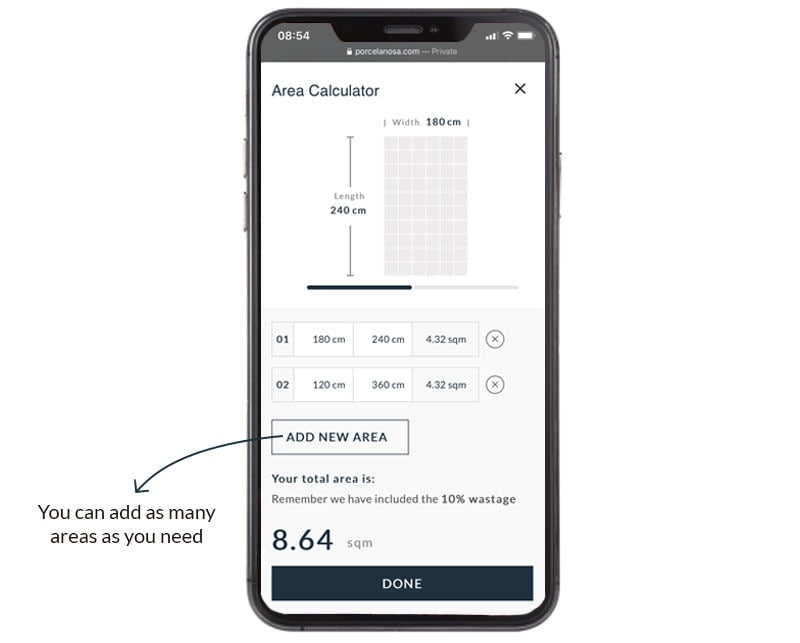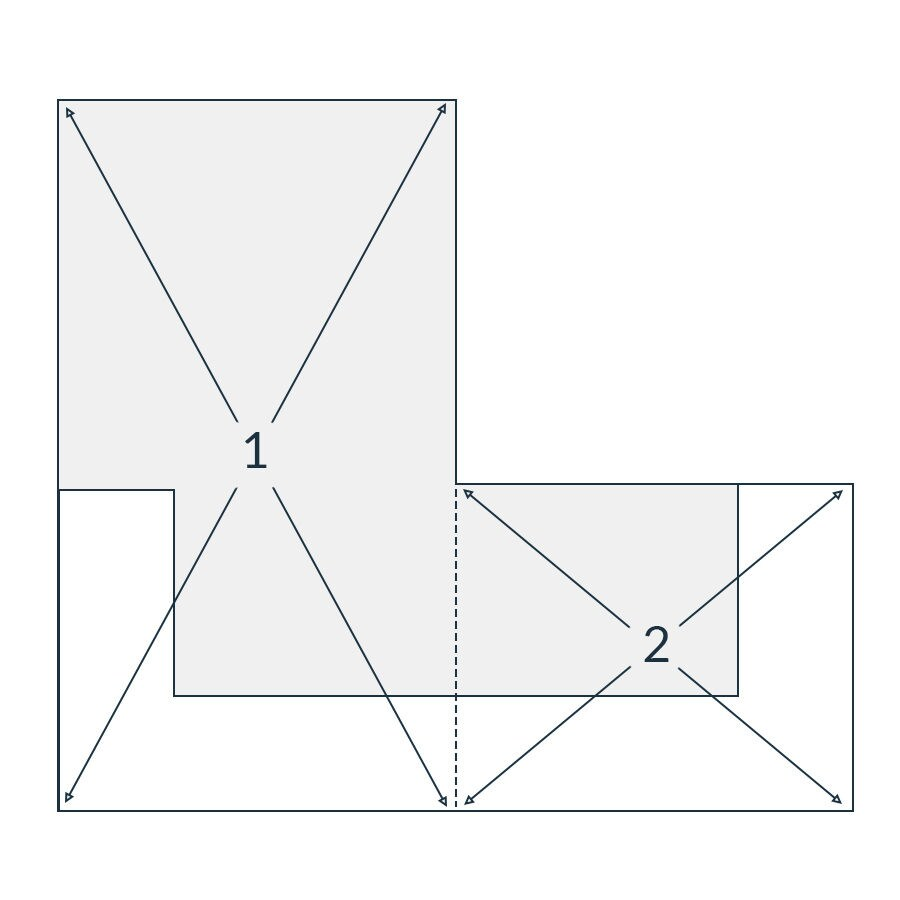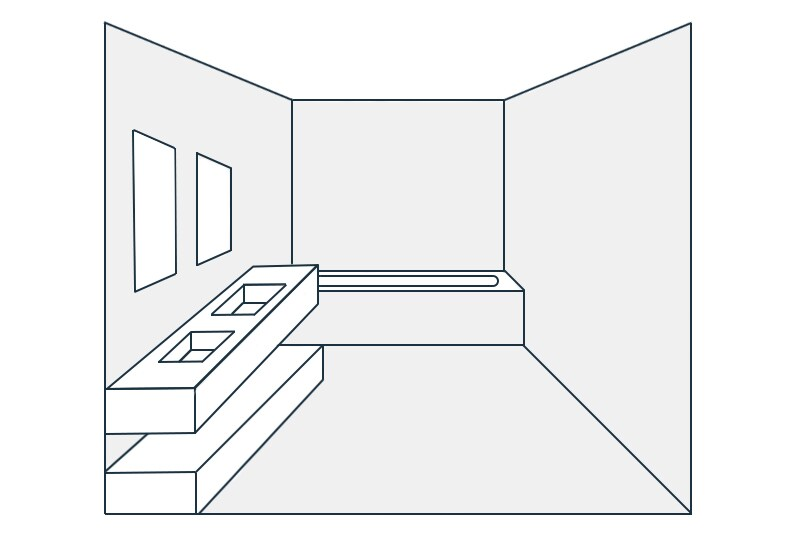October 1, 2020 | Updated: October 23, 2020

# Calculate how many tiles do you need for your project

The one mistake that is most frequent when embarking on a construction or refurbishment project is miscalculating the building budget.

When it comes to tiling there’s no reason for not being able to calculate exact quantities required. Using a square meter calculator, the number of tiles needed for a project can easily be estimated (including extras to cover wastage and damage). With this article, we’ll provide you with a rudimentary tiling calculator equally valid for wall tiles and floor tiles. And examine different types of tiles and how to calculate quantities for specific tiling projects. Remember that Porcelanosa provides an easy to use area calculator on the product page to help estimate quantities of tiles.## How do I calculate how many tiles I need?

This depends on the type of tile you are planning to use. Most wall and floor tiles are sold according by the square meter (or sq. ft.) however border tiles are sold by the linear meter (length of the longest side). And special artisan tiles are sold individually while decorative mosaics are sold in preassembled panels.

### Straightforward arithmetic tile calculator

To illustrate the number of tiles required for a typical project we will consider an area of flooring. Imagine a kitchen configured in an L shaped layout, to find out how many floor tiles are needed divide the floor into two regular rectangles. Remember to discount or subtract areas under islands and base units that are supported off the floor. To find out how many tiles will I need to measure each side multiply one side by the other and add the totals to get the overall square meters.

Rectangle One: Length x Width = Area One

Rectangle Two: Length x Width = Area Two

To calculate how many tiles needed for a room sum up all the rectangles and subtract the areas not to be tiled (under base unit cupboards).### How many tiles in a square metre

Working backwards if there is a wall tile you particularly like and know the price per square meter but want to know how many pieces in a sq.m. divide the area of the tile. Let’s say the tile measures 9cm x 6cm, divide this area into a sq.m. and you’ll have the number of separate pieces.

9cm x 6cm = 54 sq.cm. ÷ 10,000 sq.cm. = 185.19 or rounded up to 186 pieces

### Calculate how many tiles needed for a room

Remember that leaving 10% for wastage and stored replacements for future uses is recommended. Now that we know how many tiles per sq.m. we can calculate the overall total required.Total number of tiles required = (Area One + Area Two) x 1.1

## How many vinyl tiles do I need?

Vinyl tile calculation is exactly the same as any other tile with two important differences however. Vinyl tiles are easier to cut and they are sold in larger quantities, a typical box might contain sufficient coverage for 1.85 sq.m. or 20 sq.ft. for instance.

## How many mosaic tiles do I need?

Decorative mosaics are sold in self assembled panels with a backing material. This makes it easier to achieve a uniform pattern. Normally they are sold in panels of 30 x 30 cm, to cover a shower wall enclosure measuring 2.5 m in height by 1.5 m in length:

2.5 x 1.5 = 3.75 sq.m. ÷ 0.09 (area of the preassembled mosaic panel) = 41.66 or 42 panels of mosaic

### How to calculate how many tiles I need for my bathroom?

With all the different area calculations for floor and wall together with a linear measurement for the trim tile or skirting tile, we can now calculate the entire quantity of tiles required for a bathroom project.Total number of tiles required = (Σ total surface area of each wall) x 1.1